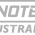## Pages

### Thermodynamics - important points to be noted for competitive exams

➔ Tephigram is the name of temperature entropy diagram

➔ PV graph in a adiabatic change is called Isentropic.

Entropy of a system is an index of “unavailable energy”.

➔ When gas is expanded, work is done by the gas on surroundings.

➔ The size of “Kelvin degree” is equal to “Centigrade”.

➔ The efficiency of a carnot engine increases by raising the temperature of the source.

➔ Work done per cycle is given by the area enclosed in the indicator diagram.

➔ Conversion of heat energy into electrical energy can be made by “Thermocouple”.

➔ f(P,V,T) =0 exists for an equilibrium state and is called equation of state.

➔ The area of cycle of T-S diagram gives the “available thermal energy for useful work” in a reversible process.

➔ Uses of TS diagram:
a) used in meteorology b) check efficiency of heat engine c) useful in predicting defects of performance of engine d) to obtain work value of fuel used.

➔ Change in entropy of universe due to free expansion is
∆S = nR log e(Vf/Vi)

➔ Loss of available of energy = To.dS where ‘To’ is lowest available temperature in system.

➔ In order to maintain a body in an isothermal condition, heat has to be either supplied or withdrawn.

➔ When a gas expands adiabatically, the temperature decreases.

➔ When a gas is compressed, the temperature increases because work is done on the gas.

➔ The work done in an adiabatic change in a particular gas depends upon only change in temperature.

➔ In an adiabatic compression, the decrease in volume is associated with increase in temperature & increase in pressure.

➔ For an isothermal expansion of a perfect gas, the value of dP/P is equal to -dV/V.

➔For an adiabatic expansion of a perfect gas, the value dP/P is equal to -𝛾dV/V.

A reversible process is always “quasi-static”, but every quasi-static process need not be a reversible process.

➔ For reversible cycle: ∆P = ∆V = ∆T = ∆U = ∆H

➔ dW = PdV is only applicable to reversible process.

➔ In case of “irreversible processes”, dW is not equal to PdV;

For free expansion, dV=0, the work may be zero (in case of PV work)

➔ Work and heat are path functions.

➔ Work is not a thermodynamic property as it is not a state function and it is not a exact differential.

➔ Both thermodynamic and temperature scales use a single reference temperature i.e triple point of water.

➔ dW = PdV is only applicable to reversible process.

➔ In case of “irreversible processes”, dW is not equal to PdV;

#### 1 comment:

1.Nice post for me and It is a very different blog than the usual ones I visit. From this post , I get more knowledge and I read a lot of interesting content here. Thanks for sharing a knowledgeable post. Thermal Monocular for Sale